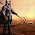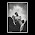## Wednesday, 13 June 2012

### CBSE - Class 9 - Maths - CH2 - Polynomials - Exercise 2.1

Q1: What is a polynomial?

Answer: A polynomial is an algebraic expression, in which no variables appear in denominators or under radical signs, and all variables that do appear are powers of positive integers.

Exercise 2.1

Q1: Which of the following expressions are polynomials in one variable and which are not? State reasons for your answer.
(i) 4x2 – 3x + 7
(ii) y2 + √2
(iii) 3√t + t√2
(iv) y +2/y
(v) x10 + y3 + t50

Answer
(i) 4x2 – 3x + 7
Because polynomial has one variable 'x', yes, this expression is a polynomial in one variable x.

(ii) y2 + √2
yes, this expression is a polynomial in one variable y.

(iii) 3√t + t√2
The exponent of variable t is ½, which is not a whole number. The expression is not a polynomial in one variable.

(iv) y +2/y
The variable has power of -ve integer, the expression is not a polynomial in one variable.

(v) x10 + y3 + t50
This expression is a polynomial in 3 variables x, y, and t. So, it is not a polynomial in one variable.

Q2. Write the coefficients of x2 in each of the following:
(i) 2 + x2 + x
(ii) 2 – x2 + x3
(iii) (π/2)x2 + x
(iv) √2.x − 1

Answer: The numerical values (including +ve or -ve signs) of the terms in a polynomial are called the coefficients of the polynomial.
(i) 2 + x2 + x
The coefficient of  x2 is 1.

(ii) 2 – x2 + x3
The coefficient of  x2 is -1.

(iii) (π/2)x2 + x
The coefficient of  x2 is π/2

(iv) √2.x − 1 = 0.x2+ √2.x − 1
The coefficient of  x2 is 0.

Q3. Give one example each of a binomial of degree 35, and of a monomial of degree 100.

Answer: Binomial of degree 35 = 3x35 + x
Monomial of degree 100 = 37t100

Note: Degree of a polynomial is the highest power of the variable in the polynomial. Monomial has only one term in it. Binomial has two terms in it.

Q4. Write the degree of each of the following polynomials:
(i) 5x3 + 4x2 +7x
(ii) 4 – y2 (iii) 5t – √7 (iv) 3

Answer: Degree of a polynomial is the highest power of variable in the polynomial.
(i) Degree of polynomial f(x) = 5x3 + 4x2 +7x  is 3.

(ii) Degree of polynomial f(y) = 4 – y2 is 2

(iii) Degree of polynomial f(t) = 5t – √7 is 1

(iv) Degree of polynomial f(x) = 3 is 0 (constant polynomial).

Q5. Classify the following as linear, quadratic and cubic polynomials:
(i) x2 + x
(ii) x – x3
(iii) y + y2 + 4
(iv) 1 + x
(v) 3t
(vi) r2
(vii) 7x3

Answer
Degree of linear polynomial = 1
Degree of quadratic polynomial = 2
Degree of cubic polynomial = 3
(i) x2 + x  is a quadratic polynomial (degree = 2)
(ii) x – x3 is a cubic polynomial. (degree = 3).
(iii) y + y2 + 4 is a quadratic polynomial (degree = 2)
(iv) 1 + x is linear polynomial (degree =1 )
(v) 3t is a linear polynomial (degree =1 )
(vi) r2 is a  quadratic polynomial (degree = 2)
(vii) 7x3 is a cubic polynomial. (degree = 3).

Q6: What is the degree of a non-zero constant polynomial?

Answer: Zero

Q7: Polynomial having three terms are called as ____.

Answer: Trinomials.

Q8: What is the degree of the zero polynomial?

Answer: The degree of the zero polynomial is not defined.

Q9: The constant polynomial 0 is called _____.
Answer: The zero polynomial.

Q10: What is the standard form of writing a polynomial?

Answer:
1. While writing a polynomial in standard form, term with higher terms are written first.
2. The coefficient of the first term (called leading coefficient) should be +ve.

Q11: Write the polynomial 7x2 + 8x + 6 + 5x3 in standard form.

Answer: Standard form 5x3 + 7x2 + 8x + 6.

Q12: Write the polynomial 3x2 - 8x + 6 - 5x3 in standard form.

Answer: Write with terms in decreasing degree =  -5x3 + 3x2 - 8x + 6.
Since the leading co-efficient be +ve, multiple all the terms with -1, i.e.
= -1 (-5x3 + 3x2 - 8x + 6)
= 5x3 - 3x2 + 8x - 6#### 2 comments:

1.Never thought maths so easy

1.ya you are right

We love to hear your thoughts about this post!